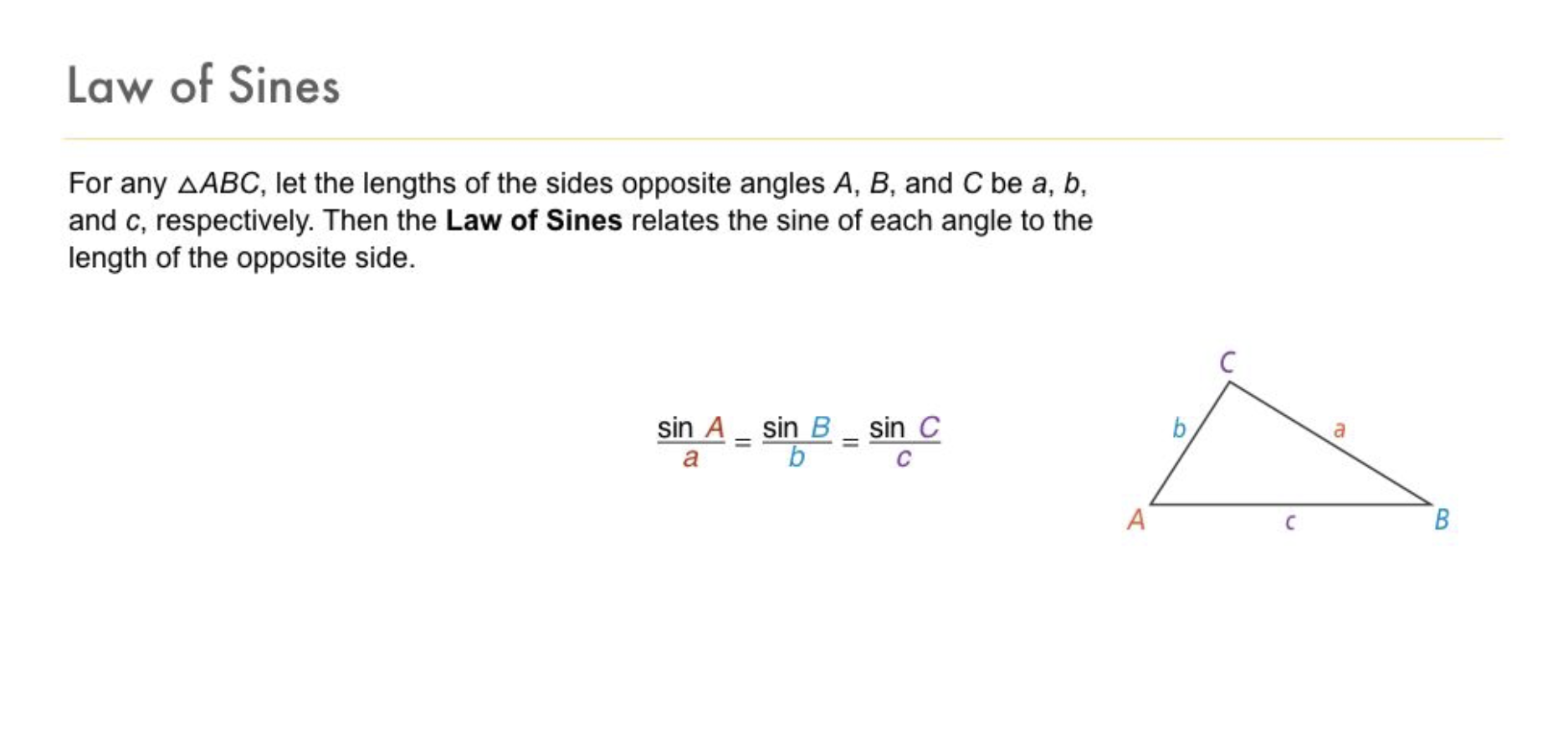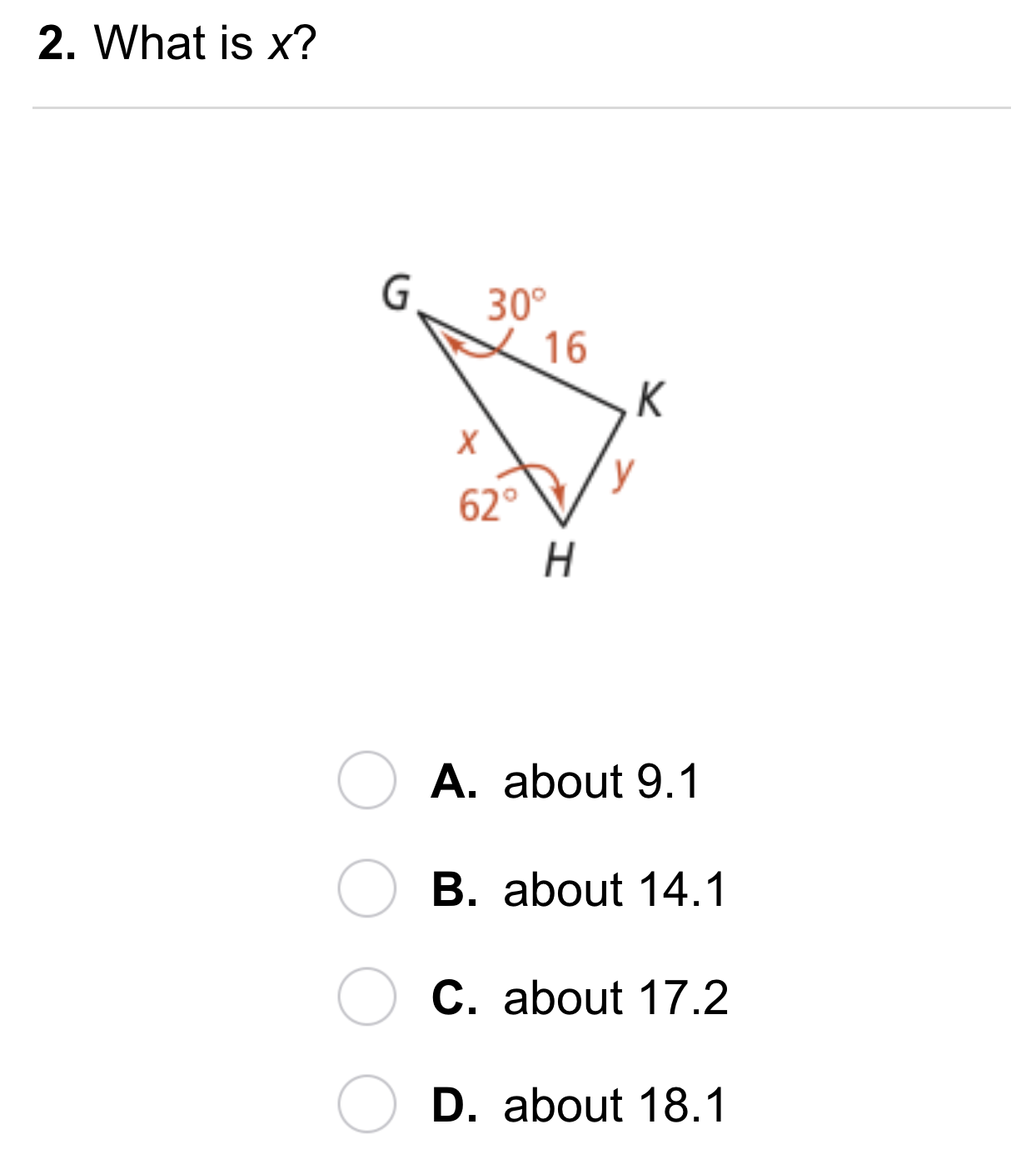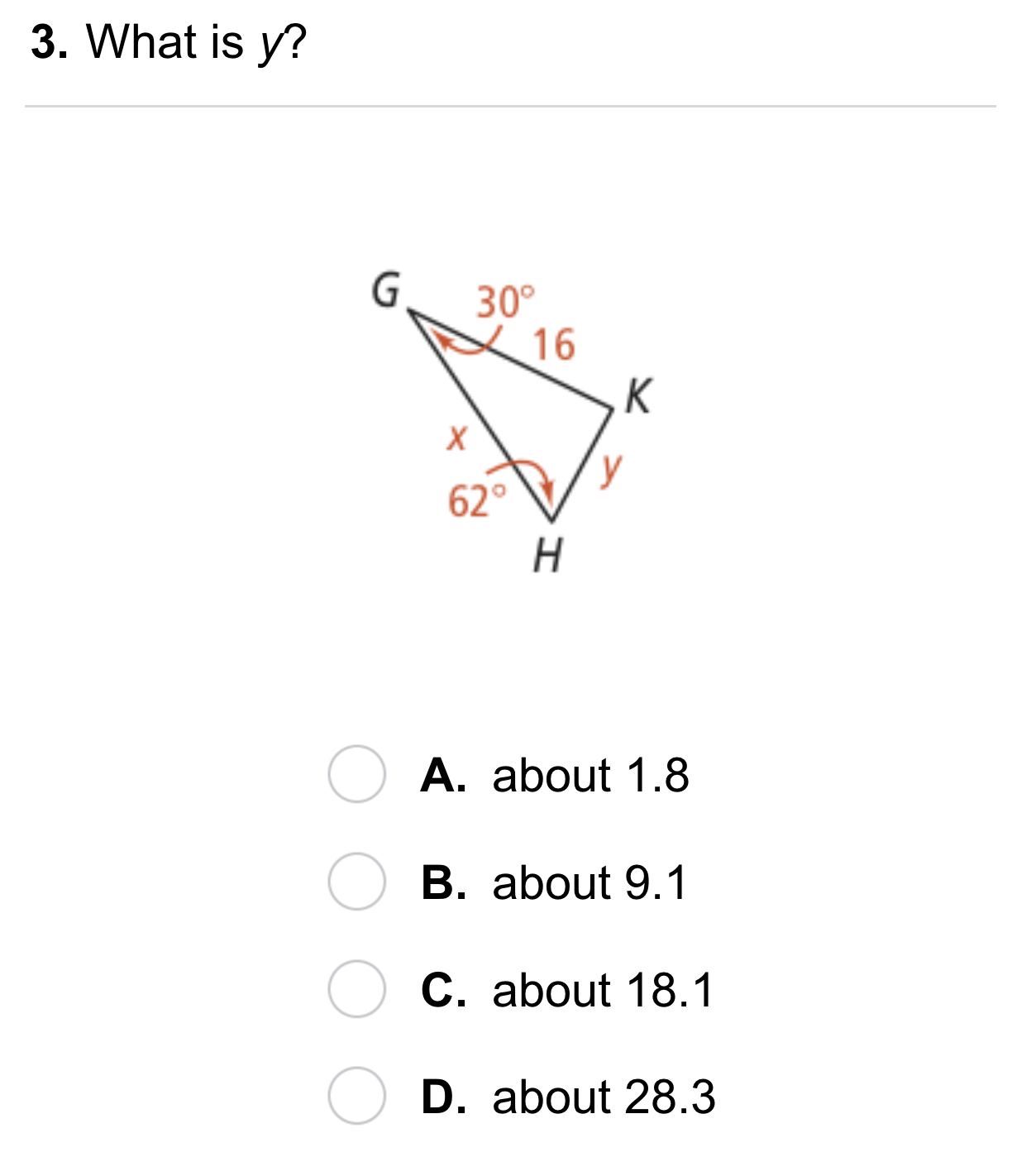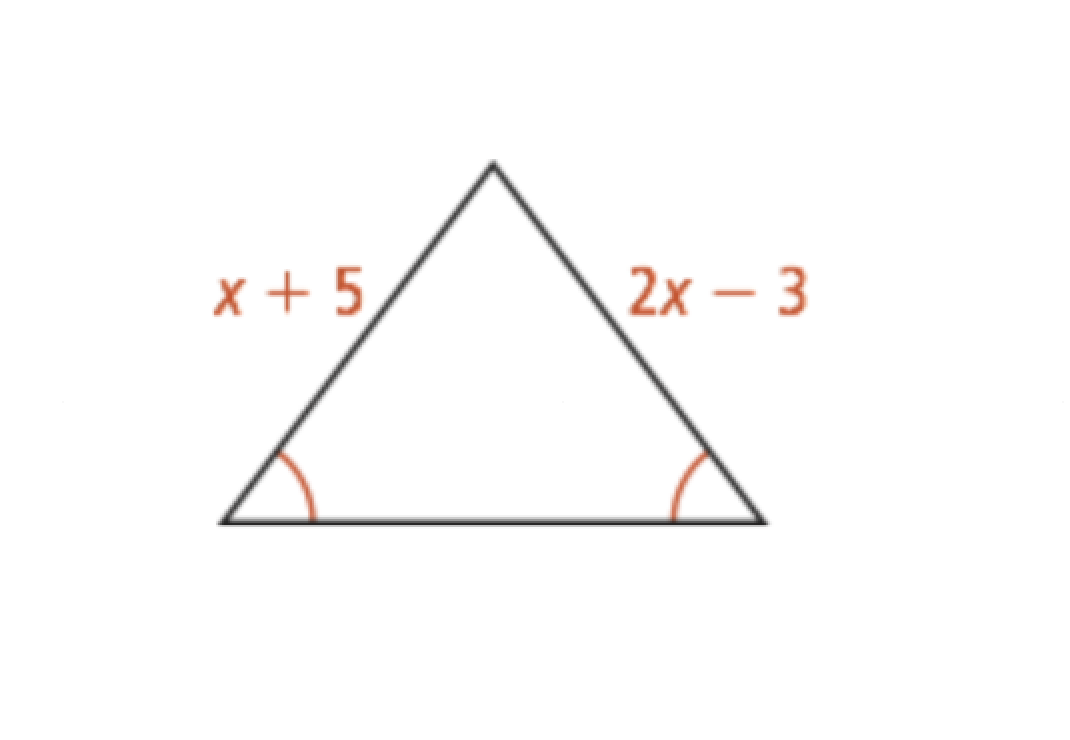Geometry 8-5 Law of Sines
starstarstarstarstarstarstarstarstarstar
by Matthew Richardson
| 21 Questions
Note from the author:
A complete formative lesson with embedded slideshow, mini lecture screencasts, checks for understanding, practice items, mixed review, and reflection. I create these assignments to supplement each lesson of Pearson's Common Core Edition Algebra 1, Algebra 2, and Geometry courses. See also mathquest.net and twitter.com/mathquestEDU.1
1
10
Solve It! A search-and-rescue boat spots a lost hiker on the edge of a rock shelf. How many feet is the boat from the hiker? Round to the nearest whole foot and enter only a number. Hint: Consider partitioning the triangle into two right triangles.2
2
10
Problem 1 Got It?
A
B
C
D3
3
10
Problem 2 Got It?
A
B
C
D4
4
10
Problem 3 Got It?
A
B
C
D5
5
10
A
B
C
D6
6
10
A
B
C
D7
7
10
A
B
C
D8
10
Reasoning: If you know the three side lengths of a triangle, can you use the Law of Sines to find the missing angle measures? Explain.
9
10
Error Analysis: In △PQR, PQ = 4 cm, QR = 3 cm, and m∠R = 75. Your friend uses the Law of Sines to write the following proporiton to find m∠P.10
10
Review Lesson 8-4: Indirect Measurement: A hot-air balloon pilot sights the landing field from a height of 2000 ft. The angle of depression is 24°. Create an illustration to model the scenario on the canvas. Remember that you can upload images to the canvas. Label all know quantities and relevant information.
11
10
Review Lesson 8-4: Refer to your work in the previous item. To the nearest foot, what is the ground distance from the hot-air balloon to the landing field? Enter only a number.12
12
10
Review Lesson 4-5: What is the value of x? Enter only a number.
You may use the embedded Desmos scientific calculator below for the Mixed Review items that follow.
13
10
Review Lesson 8-3: Evaluate the expression. Round your answer to the nearest hundredth. Include a 0 placeholder in the ones place, if needed. Enter only a number.
14
10
Review Lesson 8-3: Evaluate the expression. Round your answer to the nearest hundredth. Include a 0 placeholder in the ones place, if needed. Enter only a number.
15
10
Review Lesson 8-3: Evaluate the expression. Round your answer to the nearest hundredth. Include a 0 placeholder in the ones place, if needed. Enter only a number.
16
10
Review Lesson 8-3: Evaluate the expression. Round your answer to the nearest hundredth. Include a 0 placeholder in the ones place, if needed. Enter only a number.17
10
Vocabulary Review: Draw a line segment from each angle of the triangle to its opposite side.
18
10
Vocabulary Review: A ratio is the comparison of two quantities by __?__.
subtraction
multiplication
division19
10
Use Your Vocabulary: A triangle has a given acute angle. What is its sine ratio?

20
10
Use Your Vocabulary: A right triangle has one acute angle measuring 36.9°. The length of the side adjacent to the angle is 4 units, and the length of the side opposite this angle is 3 units. The length of the hypotenuse is 5 units. What is the sine ratio if the 36.9° angle?
4/3
3/5
5/4
5/3
4/5
21
10
Reflection: Math Success